Geology Model Test - 2017

# Geology Model Test - 2017 - IIT JAM

Test Description

## 60 Questions MCQ Test IIT JAM Past Year Papers and Model Test Paper (All Branches) - Geology Model Test - 2017

Geology Model Test - 2017 for IIT JAM 2023 is part of IIT JAM Past Year Papers and Model Test Paper (All Branches) preparation. The Geology Model Test - 2017 questions and answers have been prepared according to the IIT JAM exam syllabus.The Geology Model Test - 2017 MCQs are made for IIT JAM 2023 Exam. Find important definitions, questions, notes, meanings, examples, exercises, MCQs and online tests for Geology Model Test - 2017 below.
Solutions of Geology Model Test - 2017 questions in English are available as part of our IIT JAM Past Year Papers and Model Test Paper (All Branches) for IIT JAM & Geology Model Test - 2017 solutions in Hindi for IIT JAM Past Year Papers and Model Test Paper (All Branches) course. Download more important topics, notes, lectures and mock test series for IIT JAM Exam by signing up for free. Attempt Geology Model Test - 2017 | 60 questions in 180 minutes | Mock test for IIT JAM preparation | Free important questions MCQ to study IIT JAM Past Year Papers and Model Test Paper (All Branches) for IIT JAM Exam | Download free PDF with solutions
 1 Crore+ students have signed up on EduRev. Have you?
Geology Model Test - 2017 - Question 1

### A fault in which the displacement of limbs take place at a right angle to the surface of discontinuity (like dyke) are known as:

Geology Model Test - 2017 - Question 2

### Which sequence of the materials below shows no missing steps, and could occur during the rock cycle ?

Geology Model Test - 2017 - Question 3

### Which of the following has not been considered as an earthquake precursor ?

Geology Model Test - 2017 - Question 4

The heat that caused melting in the Earthís early history was supplied from which of the following events or causes ?

Geology Model Test - 2017 - Question 5

Referring to the diagram above, path A is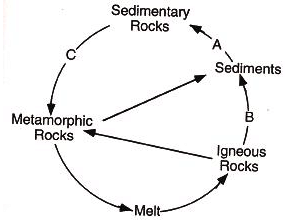Geology Model Test - 2017 - Question 6

The theory of plate tectonics was not initially widely accepted because .

Geology Model Test - 2017 - Question 7

Although igneous in origin, the chilled margins between the rounded shapes show no visible crystals. What is the name of this kind of igneous texture ?

Geology Model Test - 2017 - Question 8

The outcrop of manganese deposits exhibit color

Geology Model Test - 2017 - Question 9

Volcanism is associated with which of the following types of plate boundaries ?

Geology Model Test - 2017 - Question 10

What geological structure is shown on the map ?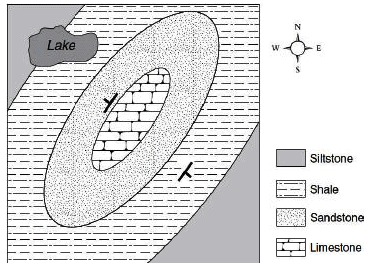Geology Model Test - 2017 - Question 11

Consider the following characteristics of a rock type :

(1) Formed by the cooling and solidifications of molten magma
(2) Molten lava when cooled formed into crystals
(3) Formed into lava plateaus, lava plains and lava shields

Which one of the following mentioned rocks posses the above characteristics ?

Geology Model Test - 2017 - Question 12

A cleavage consisting of closely spaced micro faults of fracture that divide the rock into a series of tabular bodies is known as :

Geology Model Test - 2017 - Question 13

Which type of cross- bedding cannot be used to tell top and bottom :

Geology Model Test - 2017 - Question 14

If a new planet were found between Venus and Mercury, which of the following features would it most likely have ?

Geology Model Test - 2017 - Question 15

Which labelled feature is not an unconformity ?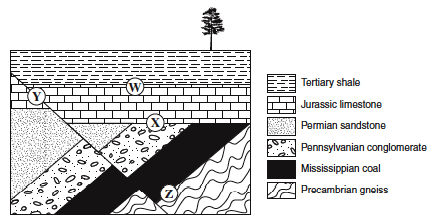Geology Model Test - 2017 - Question 16

Which of the following statements about rock deformation is false ?

Geology Model Test - 2017 - Question 17

If the sedimentary rocks on a geologic map form a zigzag pattern, the underlying structure probably consists of .

Geology Model Test - 2017 - Question 18

Laboratory experiments indicate that .

Geology Model Test - 2017 - Question 19

An igneous rock contains many one- centimetre diameter crystals of olivine. Which of the following statements about the igneous rock would be true ?

Geology Model Test - 2017 - Question 20

What is the difference between the zone of saturation and the zone of aeration of groundwater ?

Geology Model Test - 2017 - Question 21

Which of the following processes is most likely to preserve land plants as a large coal deposit?

Geology Model Test - 2017 - Question 22

What type of volcano is shown in the diagram ?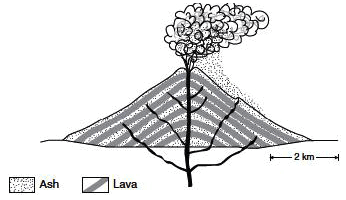Geology Model Test - 2017 - Question 23

Why is it not possible to date Pliocene fossils with the carbon- 14/nitrogen- 14 dating technique ?

Geology Model Test - 2017 - Question 24

Use the following statements about processes involved in the formation of energy resources to answer question 24.
I. Marine microorganisms die.
II. Organic material is heated and compressed.
III. Organic material is buried by sediment.
IV. Hydrocarbons sink through permeable rock because they are more dense than water.
V. Trees die in a swamp.
VI. Hydrocarbons rise through permeable rock because they are less dense than water.

Q.

Which of the following describes the order of formation of an oil deposit ?

Geology Model Test - 2017 - Question 25

How much of the original radioactive parent material would be left in a rock sample after three half- lives ?

Geology Model Test - 2017 - Question 26

According to the plate tectonic theory, which process is most likely to be occurring at the site of mountain building in the interior of a continent (e.g., the Himalayan Mountains) ?

Geology Model Test - 2017 - Question 27

Which of the following eruption and lava types is most likely produced at the Earthís surface above a subduction zone ?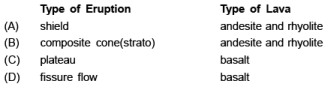Geology Model Test - 2017 - Question 28

Match the following parts of the rock cycle with the appropriate explanation.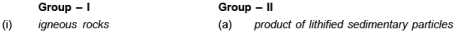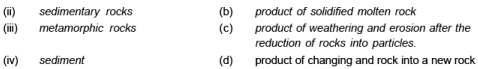Geology Model Test - 2017 - Question 29

Which of the following would be the least important factor to consider when designing an earthquake- resistant building ?

Geology Model Test - 2017 - Question 30

Which directions of compression caused the parallel alignment of the long crystals in the metamorphic rock ?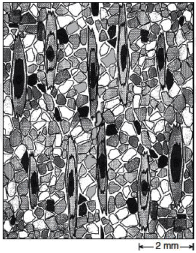*Multiple options can be correct
Geology Model Test - 2017 - Question 31

The attitude of folds and fold systems is or can be fully characterized by :

*Multiple options can be correct
Geology Model Test - 2017 - Question 32

According to the rock cycle, identify the statements which are correct _______ .

*Multiple options can be correct
Geology Model Test - 2017 - Question 33

Which of the following processes of the rock cycle are correctly matched with their explanations

*Multiple options can be correct
Geology Model Test - 2017 - Question 34

Which of the following instruments are used in geochemical prospecting ?

*Multiple options can be correct
Geology Model Test - 2017 - Question 35

Which of the following are closing ocean basin ?

*Multiple options can be correct
Geology Model Test - 2017 - Question 36

Which of the following are the most abundant elements in nebula (gas clouds) in the universe ?

*Multiple options can be correct
Geology Model Test - 2017 - Question 37

In general, igneous rocks :

*Multiple options can be correct
Geology Model Test - 2017 - Question 38

Which of the following features are associated with a convergent plate boundary ?

*Multiple options can be correct
Geology Model Test - 2017 - Question 39

What caused dust and condensing material to accrete into planetesimals ?

*Multiple options can be correct
Geology Model Test - 2017 - Question 40

Nappe structures can be formed by :

*Answer can only contain numeric values
Geology Model Test - 2017 - Question 41

As a result of radioactive decay of an isotope, _____ percent original parent isotope remains after four half- lives ?

*Answer can only contain numeric values
Geology Model Test - 2017 - Question 42

Locations S and T shown in the southwest corner of the map are 500 km apart. _____ will be the distance between them change in one year ?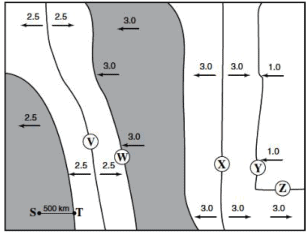*Answer can only contain numeric values
Geology Model Test - 2017 - Question 43

On this topographic map, the contour interval is ______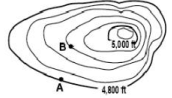*Answer can only contain numeric values
Geology Model Test - 2017 - Question 44

The average gravitational force of the earth is ______cm/s2.

*Answer can only contain numeric values
Geology Model Test - 2017 - Question 45

The difference between the equatorial and polar diameters of the Earth is _____

*Answer can only contain numeric values
Geology Model Test - 2017 - Question 46

Though many beds are upright others are not. For example, an overturned bed is one that has been rotated more than __________ degrees.

*Answer can only contain numeric values
Geology Model Test - 2017 - Question 47

The time taken for light from the sun to reach the earth in seconds is_____

*Answer can only contain numeric values
Geology Model Test - 2017 - Question 48

The archaen greenstone belts range in age from______M.Y

*Answer can only contain numeric values
Geology Model Test - 2017 - Question 49

There are 48 different forms in crystals. Of these, the cubic system has forms.

*Answer can only contain numeric values
Geology Model Test - 2017 - Question 50

Silicon is the second most abundant element in the earthís crust after oxygen. It occupies ______ % of the total volume of the crust.

*Answer can only contain numeric values
Geology Model Test - 2017 - Question 51

Two ions can replace each other in a crystal structure only if the difference between their ionic radii does not exceed ________ %.

*Answer can only contain numeric values
Geology Model Test - 2017 - Question 52

The optimum temperature under which the reef- building corals thrive ranges from ________ ºC.

*Answer can only contain numeric values
Geology Model Test - 2017 - Question 53

The ratio of FeO:Cr2O3 in chromite is ________ .

*Answer can only contain numeric values
Geology Model Test - 2017 - Question 54

The great bulk of Banded iron formations (BIFs) was laid down during the time intervals of M.Y.

*Answer can only contain numeric values
Geology Model Test - 2017 - Question 55

The Fe+2/Mg ratio in ortho and clinopyroxenes can be used as a geothermometer. This is given as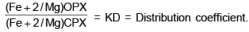The distribution coefficient for igneous rocks is_______

*Answer can only contain numeric values
Geology Model Test - 2017 - Question 56

In the statement “ Indian sub-continent which formed a part of the Gondwana Supercontinent separated out around X million years before the present”, ‘X’ stands for______

*Answer can only contain numeric values
Geology Model Test - 2017 - Question 57

In the case of diamond drilling, the core that is recovered in sticks longer than 10 cm is expressed as a percentage of the rock quality designation (RQD. High quality materials have RQD values greater than _____

*Answer can only contain numeric values
Geology Model Test - 2017 - Question 58

The main Unonformity separating the Aechaen and Proterozoic successions occur at ________ M.Y.

*Answer can only contain numeric values
Geology Model Test - 2017 - Question 59

The wavelength of the F- Fraunhofer line is ________ .

*Answer can only contain numeric values
Geology Model Test - 2017 - Question 60

The mineral specimen shown in the photograph can be scratched by a copper penny and fizzes in acid. Its hardness is ______ .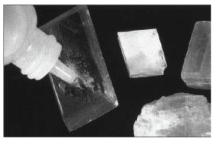## IIT JAM Past Year Papers and Model Test Paper (All Branches)

8 docs|48 tests
Information about Geology Model Test - 2017 Page
In this test you can find the Exam questions for Geology Model Test - 2017 solved & explained in the simplest way possible. Besides giving Questions and answers for Geology Model Test - 2017, EduRev gives you an ample number of Online tests for practice

## IIT JAM Past Year Papers and Model Test Paper (All Branches)

8 docs|48 tests(Scan QR code)